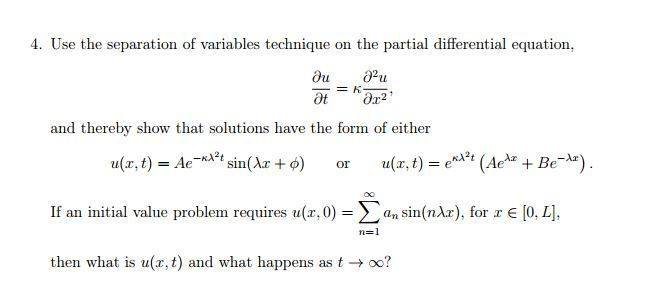# Separation of variables PDE

## Homework Statement## The Attempt at a Solution

I managed to do the first part of the question. But i'm not sure how to find u(x,t) with that initial condition.

The solution says; "since ##u(x,0) = \sum_{n=1}^\infty a_{n}\sin{(n\lambda x)}## Then it follows by linearity that ##u(x,t)=\sum_{n=1}^\infty a_{n}\sin{(n\lambda x)}e^{-n^2\lambda^{2}kt}##"
Could somebody please explain how the initial condition implies this. Thank you.

haruspex
Homework Helper
Gold Member
2020 Award
From the reference to linearity, I think the argument is that you can consider the set of solutions un(x, t) to the equation, the nth one having the initial condition un(x, 0) = ##a_n\sin(n \lambda x)##. Then sum them to get the solution to the summed initial condition.

BvU
Homework Helper
please explain how the initial condition implies this
makes me suspect pondzo is still struggling with an insight: that each and every term in ##u(x,0) = \Sigma ... ## is of a form that satisfies the differential equation at t=0: ##A##, ##\lambda## and ##\phi## are integration constants.

(I assume the linearity is not a problem: if ua and ub are solutions, then C ##\times## ua + D ##\times## ub is also a solution)

If a particular problem requires the integration constants to have values like ##a_n##, ##n\lambda## and ##0##, respectively, that's fine: they are still solutions. And for each of these solutions the time development is known.

I don't think it is a problem with the concept of linearity, I understand that (at least I think I do). And i realize that the A, lambda and phi are integration constants. My problem is that i can't see how that initial condition implies (by linearity) that in the separation of variable technique the time dependence is of the form ##e^{-n^2\lambda^{2}kt}## In particular I don't know where the n^2 comes from. Maybe I am missing something blindingly obvious, after all we have only just been introduced to the wave equation and how one can approach solving special cases of it.

haruspex
Homework Helper
Gold Member
2020 Award
I don't think it is a problem with the concept of linearity, I understand that (at least I think I do). And i realize that the A, lambda and phi are integration constants. My problem is that i can't see how that initial condition implies (by linearity) that in the separation of variable technique the time dependence is of the form ##e^{-n^2\lambda^{2}kt}## In particular I don't know where the n^2 comes from. Maybe I am missing something blindingly obvious, after all we have only just been introduced to the wave equation and how one can approach solving special cases of it.
I think you are being thrown by the reuse of lambda with, effectively, a different meaning. To make it clearer, let's use a different letter for the constant of integration: ##u(x,t)=A\sin{(\mu x)}e^{-\mu^{2}\kappa t}##
And let's simplify it by saying the initial condition is just ##u(x,0) = \sin{(n\lambda x)}##, where now lambda is a given constant. What do you have to substitute for ##\mu## in the u(x,t) formula to apply the initial condition?

Ok I think i finally get it.
The first part of the question required us to prove that solutions to this PDE had the form (when using sep var);
##u(x,t)=Ae^{-k\lambda^2 t}\sin{(\lambda x+\phi)}##
Then we were given an initial condition: ##u(x,0) = \sum_{n=1}^\infty a_{n}\sin{(n\lambda x)}##
And in order to apply this initial condition I need to let the lambda in the first equation equal ##n\lambda## which would yield the given solution.
I have one more question though, the phase constant in the sine argument of the final solution form is zero. Is this because the phase constant is zero in the initial condition (sorry if that seems a trivial question to you)?

haruspex
Homework Helper
Gold Member
2020 Award
Ok I think i finally get it.
The first part of the question required us to prove that solutions to this PDE had the form (when using sep var);
##u(x,t)=Ae^{-k\lambda^2 t}\sin{(\lambda x+\phi)}##
Then we were given an initial condition: ##u(x,0) = \sum_{n=1}^\infty a_{n}\sin{(n\lambda x)}##
And in order to apply this initial condition I need to let the lambda in the first equation equal ##n\lambda## which would yield the given solution.
I have one more question though, the phase constant in the sine argument of the final solution form is zero. Is this because the phase constant is zero in the initial condition (sorry if that seems a trivial question to you)?
Yes, and yes (but 'no' to the 'is it trivial?').

Sorry i have one more question.
When solving the wave equation using separation of variables, the only interesting non trivial case is when the separation constant is negative. With a PDE like this one, can we assume the separation constant is negative or must we investigate the other cases too?

haruspex